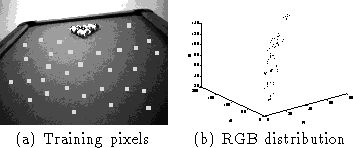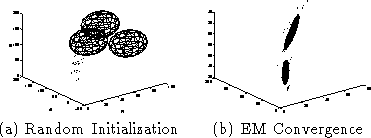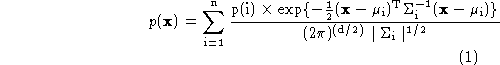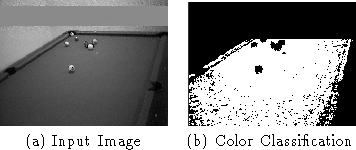Next: Contour Computation Up: Vision Processing Previous: Vision Processing

## Color Feature DetectionFigure: The color training samplesFigure: Fitting a Gaussian Mixture

To find the pool table, we train a probabilistic color model of the green felt that covers it  . This is done by taking multiple training samples of several images of a pool table under many imaging situations (offline). Each pixel in this distribution forms a 3 element vector, [R G B] which corresponds to the red, green and blue components of the color. We perform clustering on this distribution of pixels which is shown in Figure. The clustering uses Expectation Maximization (EM) to find a probability distribution model for pool table colors  . This model is a mixture of Gaussians (the appropriate number of Gaussians is determined a priori with cross-validation). The EM algorithm iterates by adjusting the parameters of the Gaussian probability model to maximize the likelihood of the training samples. The probability distribution model starts off in a random configuration and converges to the configuration in Figure. The mixture model is described by Equationwhereis an (R,G,B) vector.When a new image is acquired, the likelihood of each pixel is evaluated using this model and if it is above a threshold of probability, it is labeled as a piece of the pool table. Then, a connected component analysis is used to gather adjacent green pixels to determine larger regions of grouped table pixels in the image. This process is demonstrated in Figure. The largest connected region of green pixels is selected as the table top and proceeds through the algorithm for further processing.Figure: Pool Table Localization

Tony Jebara
Wed Feb 18 18:52:15 EST 1998